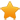Switch Editions?
CancelChannel: CodeSection,代码区,网络安全 - CodeSec
Viewing all articles

# Visualizing multiple dimensions of Google Analytics in R

0
0

(This article was first published on Tatvic Blog R , and kindly contributed toR-bloggers)

Stack bar chart It is used when we wish to visualize a combination of categorical variables ggplot(data, aes(date, fill = region)) + geom_bar()+ labs(title = "Stacked Bar Chart", x = "Date", y = "Count of Regions") where date : ga:date and region : ga:region Example ggplot(train, aes(Outlet_Location_Type, fill = Outlet_Type)) + geom_bar()+ labs(title = "Stacked Bar Chart", x = "Outlet Location Type", y = "Count of Outlets")

Heat Map

It uses intensity (density) of colors to display relationship between two or three or many variables in a two dimensional image.

It allows us to explore two dimensions as the axis and the third dimension byintensityof color. ggplot(data, aes(date, region)) + geom_raster(aes(fill = transactions))+ labs(title ="Heat Map", x = "Date", y = "Region")+ scale_fill_continuous(name = "Transactions") where date : ga:date, region : ga:region and transactions : ga:transactions

Example

ggplot(train, aes(Outlet_Identifier, Item_Type)) + geom_raster(aes(fill = Item_MRP)) + labs(title ="Heat Map", x = "Outlet Identifier", y = "Item Type") + scale_fill_continuous(name = "Item MRP")

The post Visualizing multiple dimensions of Google Analytics in R appeared first on Tatvic Blog .

Viewing all articles

More Pages to Explore .....